A system is taken from state A to state B along two different paths 1 and 2. If the heat absorbed and work done by the system along these two paths are  respectively, then

(1) ${Q}_{1}={Q}_{2}$

(2) ${W}_{1}={W}_{2}$

(3) ${Q}_{1}-{W}_{1}={Q}_{2}-{W}_{2}$

(4) ${Q}_{1}+{W}_{1}={Q}_{2}+{W}_{2}$

Concept Questions :-

First law of thermodynamics
NEET 2021 - Achiever Batch - Aryan Raj Singh

Difficulty Level:

In given process dW = 0, dQ < 0 then for a gas

1.  Temperature -  increases

2.  Volume - decreases

3.  Pressure - decreases

4.  Pressure - increases

Concept Questions :-

Types of processes
NEET 2021 - Achiever Batch - Aryan Raj Singh

Difficulty Level:

A given mass of gas expands from state A to state B by three paths 1,2 and 3 as shown in the figure. If respectively be the work done by the gas along the three paths then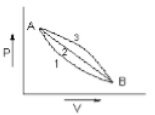1.

2.

3.

4.

Concept Questions :-

Work done by gas
NEET 2021 - Achiever Batch - Aryan Raj Singh

Difficulty Level:

The ratio of the relative rise in pressure for adiabatic compression to that for isothermal compression is

(1) $\gamma$

(2) $\frac{1}{\gamma }$

(3) 1-$\gamma$

(4) $\frac{1}{1-\gamma }$

Concept Questions :-

Types of processes
NEET 2021 - Achiever Batch - Aryan Raj Singh

Difficulty Level:

A sink, that is the system where heat is rejected, is essential for the conversion of heat into work. From which law the above inference follows?

(1) zeroth

(2) First

(3) Second

(4) Third

Concept Questions :-

Second law of thermodynamics
NEET 2021 - Achiever Batch - Aryan Raj Singh

Difficulty Level:

For the indicator diagram given below to select the wrong statement.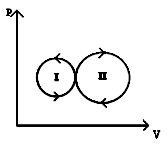1.  Cycle - II is a heat engine cycle

2.   Net work is done on the gas in cycle - I

3.   Work done is positive for the cycle I

4.  Work done is positive for the cycle - II

Concept Questions :-

Work done by gas
NEET 2021 - Achiever Batch - Aryan Raj Singh

Difficulty Level:

The variation of pressure versus temperature of an ideal gas is shown in the given diagram. From this diagram, one can conclude that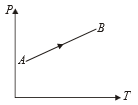1.  Volume increases continuously

2.  Volume decreases continuously

3.  Volume first increases then decreases

4.  Volume first decreases, then increase

Concept Questions :-

Types of processes
NEET 2021 - Achiever Batch - Aryan Raj Singh

Difficulty Level:

The variation of density (p) of gas with its absolute temperature (T) at constant pressure is best represented by the graph

1.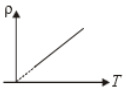2.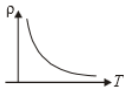3.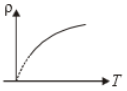4.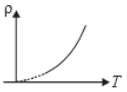Concept Questions :-

Types of processes
NEET 2021 - Achiever Batch - Aryan Raj Singh

Difficulty Level:

An ideal gas with adiabatic exponent y is heated at constant pressure and it absorbs Q heat. What fraction of this heat is used to perform external work?

1.  $\mathrm{\gamma }$

2.  $\frac{1}{\mathrm{\gamma }}$

3.

4.

Concept Questions :-

Types of processes
NEET 2021 - Achiever Batch - Aryan Raj Singh

Difficulty Level:

A Carnot engine working between 400K and 800K has a work output of 900J per cycle. The amount of heat energy supplied to engine from the source per cycle is

1.  900 J

2.  1800 J

3.  450 J

4.  2700 J

Concept Questions :-

Heat engine and refrigerator